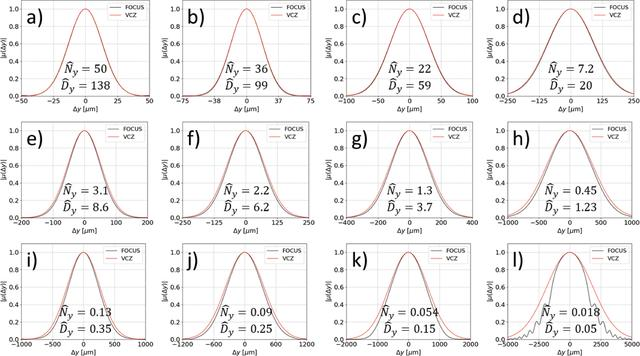disable zoom     view article Figure 4 Systematic study of the vertical coherence for a typical third-generation synchrotron light source for radiation tuned at the harmonic h = 7 with λ = 0.1 nm (a,e,i), h = 5 with λ = 0.14 nm (b,f,j), h = 3 with λ = 0.23 nm (c,g,k), and h = 1 with λ = 0.7 nm (d,h,l), and for decreasing vertical beam size σy = 40 µm (a,b,c,d), σy = 10 µm (e,f,g,h), and σy = 2 µm (i,j,k,l). Predictions based on the van Cittert and Zernike theorem (VCZ) are also reported for direct comparison. The electron beam divergence is varied according to= σy/βy where βy = 1.2 m is the vertical beta function at the undulator center. In each plot we also report the corresponding normalized parametersandaccording to equation (11).JOURNAL OFSYNCHROTRONRADIATION
ISSN: 1600-5775
Volume 30| Part 1| January 2023| Pages 217-226